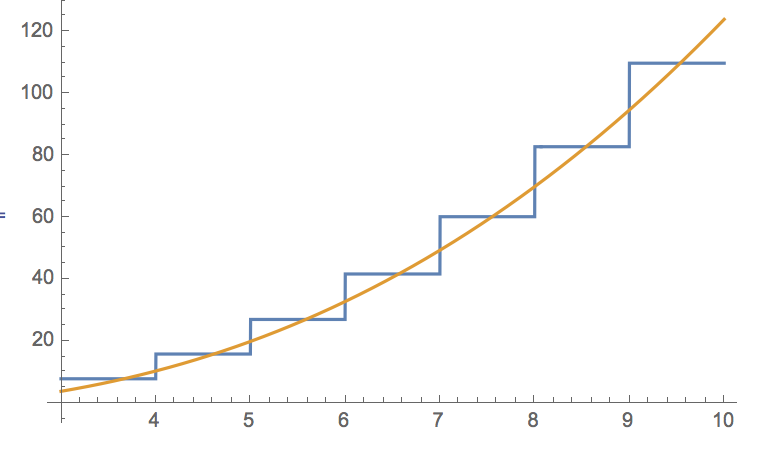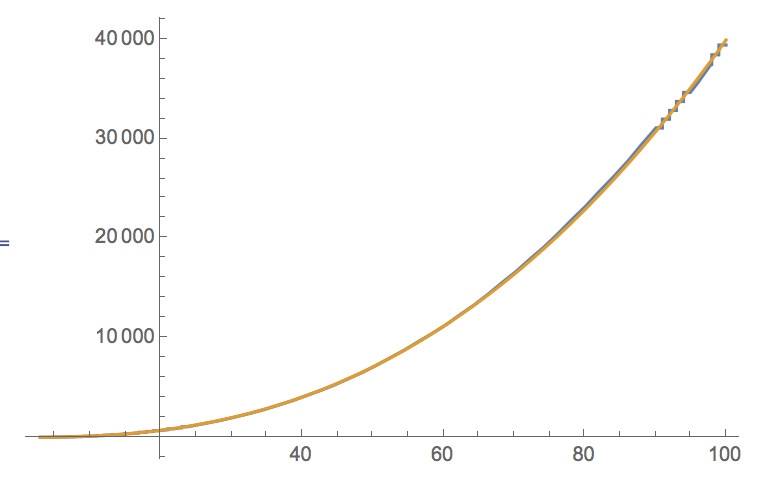# Summating divergent series arising from the application of the Euler-Maclaurin formula to power law functions with non-integer exponents

As the title says, I'm stuck trying to find an expression for $\sum_{n=a}^b n^{q}$, with q being a positive rational number but not an integer, which does not demand unfeasibly long computation times for large ranges of $b-a$. From the application where this problem originated from, the limitation $1<a<b$ applies, it is acceptable to set $a=2$ and calculate for higher starting values by subtraction, and $q=1.5$, though it may be more interesting to investigate this issue in general.

Applying the Euler-Maclaurin formula to this sum yields: $$\sum_{n=a}^b n^q = \frac{(b^{q+1}-a^{q+1})}{q+1}+\frac{(b^{q}+a^{q})}{2}+\sum_{k=1}^\infty \left(\frac{B_{2k}}{(2k)!}*(b^{q+1-2k}-a^{q+1-2k})*\prod_{i=1}^{2k-1}(q+1-i)\right)$$ for all non-integer $q$,where $B_{2k}$ are the even Bernoulli numbers.

The issue is that the series arising here is divergent, with alternating signs and increasing absolute values.

Subtracting the first two terms of the right hand side of the equation from the computed sum for which we sought an expression to begin with allows to summate this divergent series numerically. For $a=2$ and $q=1.5$, a pretty good curve fit for this difference is $0.166*b^{0.478}-1$ for up to about a million with $R^2=0.994$, but the behavior changes for even bigger values of b.

Partial sum development for the divergent series does not yield satisfying results either, any partial sum deteriorates the result compared to leaving it out entirely and only have the first two terms of the right hand side of the equation evaluated.

Since the computation time for the entire sum gets unfeasibly long for really large values of b and the numerical calculation of the sum does not allow to draw any rational conclusions in respect to the optimization problem in which this sum came up in the first place, I'd like to apply a proper summation method for divergent series to evaluate this difference.

Unfortunately the underlying sequence is obviously quite complicated and the Bernoulli numbers are difficult to account for with general methods.

Therefore, I have to ask for help with this issue, be it for the specified case with $q=1.5$ and $a=2$ or the general case if something can be said about the latter.

Which summation method is best applicable to the aforementioned divergent series?

What solution would it yield?

If that's not possible, could you propose an approximation formula for this difference whose best fit parameters will not change for values of b lying outside the range from which the numerical support of the fit was drawn, i.e. which adequately reflects the influence of the covered range of b on the value of this difference?

Help with this would be highly appreciated. Thank you in advance!

The approximation $$\sum_{n=2}^b n^{1.5} \approx \frac{8 b^{7/2}}{5(4b-5)}$$ seems very accurate. Here is the approximate relative error for various values of $b$.

$b=10$: $~~~~~0.02$
$b=10^2$: $~~~0.00015$
$b=10^3$: $~~~1.33\times 10^{-6}$
$b=10^4$: $~~~1.28\times 10^{-8}$
$b=10^5$: $~~~1.25\times 10^{-10}$
$b=10^6$: $~~~1.25\times 10^{-12}$
$b=10^7$: $~~~1.25\times 10^{-14}$

So experimentally the relative error is $O(b^{-2})$. I didn't manage to get a short expression that precise by truncating the E-M expansion.

Proof? You want proof?

• This is the first two terms $(2/5)b^{5/2}+(1/2)b^{3/2}$ from the OP's formula, up to corrections $O(b^{1/2})$. – Christian Remling Sep 27 '15 at 13:21
• Yes, you are right. So why doesn't OP use it? – Brendan McKay Sep 27 '15 at 13:49
• That's an interesting approximation, I've been comparing the resulting difference to the exact computation with that of the first two terms + power law curve fit mentioned in my OP, and while this method seems a bit worse for lower values (up to about $b=10^6$) it does catch up beyond that, likely due to the best fit of the parameters rounded to three digits. But I'm impressed that this approximation works so well with such smooth, rational numbers. Could you please explain how you came up with it? I can't quite track it right now. – MarioVX Sep 27 '15 at 18:56
• @MarioVX I found it experimentally, but Christian is right that the relative error $O(1/b^2)$ follows from the E-M expansion. Note that the E-M expansion usually diverges, but there are several rigorous bounds on the remainder after a finite number of terms. If you apply it to the number of terms for which the remainder bound is least, you will find it is quite accurate and you will have explicit bounds on the exact value. – Brendan McKay Sep 28 '15 at 0:34

[update] After some computations and explorations I've now finetuned that procedure so that I get with the same configuration as below (using the first 128 nonzero (=256 total) Bernoulli-numbers in my original answer) 30 to 50 digits precision instead of 13 digits (if we have big $b$ so that the replacement of the direct summation by the E-ML-formula is at all required) . Using the first 32 nonzero Bernoulli-numbers I get already ~20 digits precision.

If this is of practical interest I can post the Pari/GP or pseudocode.
[end update]

Usually I do this using a matrix-method for divergent summation (I think it's Noerlund summation), see the example in Pari/GP:

[q=1.5,a=2,b=10]
res = sum(k=a,b,k^q)             \\ see the expected result
\\ ~  141.6723106687563


Then with the E-M-formula I get the first two terms

s1 =  (b^(q+1)-a^(q+1))/(q+1)   + (b^q+a^q)/2
\\ ~ 141.4539665701532


Now I get the vector of E-M terms involving the Bernoulli-numbers; we'll need 128 terms for the convergence of the display to 12 digits:

n=128
tmp=vectorv(n,k, bernfrac(2*k)/(2*k)!*(b^(q+1-2*k)-a^(q+1-2*k))*prod(i=1,2*k-1,(q+1-i))))


Doing the Noerlund-summation method needs only the left-multiplication by a matrix, which is computed by two parameters $o_1=2,o_2=1$ (which I had to adapt to optimal values for the parameters $q$ and $b$ of the problem)

partsums = NoerlundSum(2.0,1.0,n) * tmp
s2=partsums[n]       \\ get the 128'th partial sum
err = s1+s2 - res    \\ test the difference to the result
\\ ~ 1.19587246024 E-13


The partial sums look like

  0.2185080122244105 at k=0
0.2184241761270169 at k=1
0.2183888314589473 ...
0.2183711219347356
0.2183613058497571
...
0.2183440986032484
0.2183440986032327
0.2183440986032185
0.2183440986032056
0.2183440986031940    at k=127


For $b=100$ I get

res ~ 40500.22451531894
s1  ~ 40499.15147186258
s2  ~     1.073043456362870
err = s1+s2 - res ~  1.627013113495834 E-13


with the partial sums looking like

  1.073223304703363  at k=0
1.073131493924563  at k=1
1.073092733636016
1.073073283966398
...
1.073043456362943
1.073043456362922
1.073043456362903
1.073043456362886
1.073043456362870 at k=127


For $b=10000$ I get

  res ~  4000500011.474515
s1  ~  4000499999.151472
s2  ~          12.32304293605502
err = s1+s2 - res ~  1.642705338479837 E-13


and the partial sums are

  12.32322330470336
12.32313123376831
12.32309236198489
...
12.32304293605512
12.32304293605509
12.32304293605507
12.32304293605505
12.32304293605504
12.32304293605502


For $b=10\, 000\, 000$ I get wit the same size of error of about $1e-13$

 res ~ 126491122218123868.381074720929
s1  ~ 126491122218123473.273324264348
s2  ~                395.107750456581621235495698141
err ~                                1.64272106388128874739559751446 E-13


Note also, that the error is given as absolute error, so this is different from (more exact than) the result in @BrendanMcKay 's short formula when $b$ increases even more.

So in general I think, some divergent-summation method like Borel-summation might even be more efficient to sum the vector/sequence of terms to even more precision than gotten by this "Noerlund"-matrix and 128 terms.

I would just approximate this sum $$\sum_{n=2}^b n^{1.5}$$ by the integral $$\int_2^b x^{1.5}\,dx=(b^{2.5}-2^{2.5})/2.5$$, the result is quite accurate even for small values of $$b$$ see the plots (for $$b$$ up to 10 and up to 100):• But how accurate is it for large values of $b$? OP already has something "pretty good" for $b$ up to a million, but is concerned with deteriorating accuracy beyond that. – Gerry Myerson Sep 26 '15 at 23:13
• On each interval $(n,n+1)$, you're off by $\sim n^{1/2}$, so the total absolute error is $\sim b^{3/2}$. – Christian Remling Sep 27 '15 at 0:30
• The integral is already included in the Euler-Maclaurin formula as the first term of the right hand side of the equation I posted. It's an insufficient approximation to the sum for two reasons: 1) The first and last columns' outer halfs are not considered, this is corrected in the formula through the second term on the right hand side. 2) The positive and negative area differences between the steps and the continuous function do not eliminate each other for all functions, in particular not for the non-integer exponent power law functions investigated here. Hence the divergent series. – MarioVX Sep 27 '15 at 1:49
• well, I guess it would depend on the application whether or not the $1/b$ relative accuracy of the integral approximation to the sum is acceptable or not, but quite generally I would think that, when confronted with a divergent (aysmptotic) series approximation it makes sense to stop at the first (or first few) terms. In any case, in response to @GerryMyerson, the accuracy of the integral approximation remains of relative order $1/b$ no matter how large $b$, it does not deteriorate. – Carlo Beenakker Sep 27 '15 at 11:14
• You are correct that the relative error doesn't increase, but the absolute error does. I started with an approximation already a lot more concise than the integral and sought to improve that further though, so this is a step back. Thank you for the figures though, as the left one illustrates quite well how the area differences don't eliminate (one can tell by the eye the positive and negative difference areas are different in size), and hence where the accumulating deviation comes from. – MarioVX Sep 27 '15 at 18:02